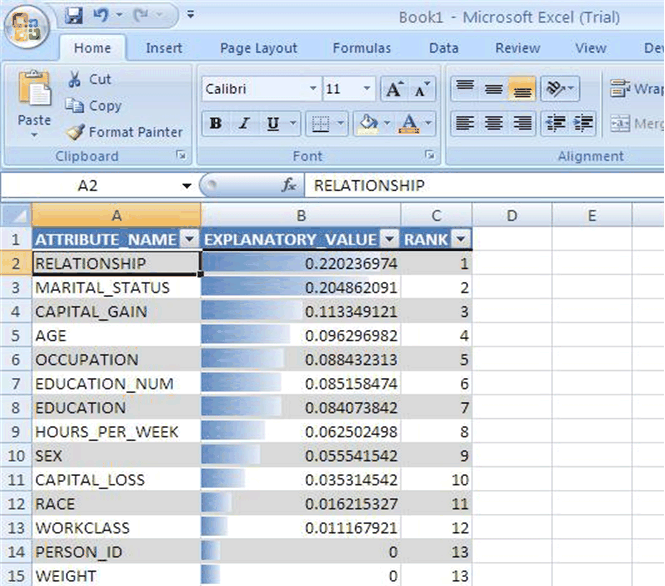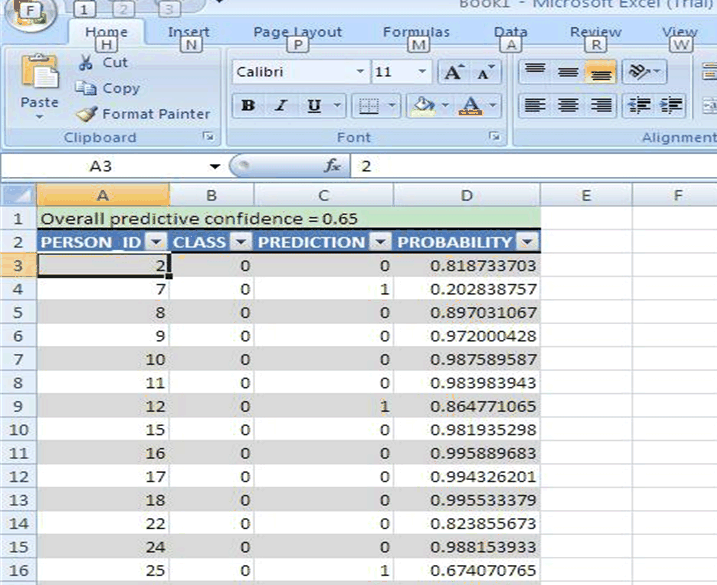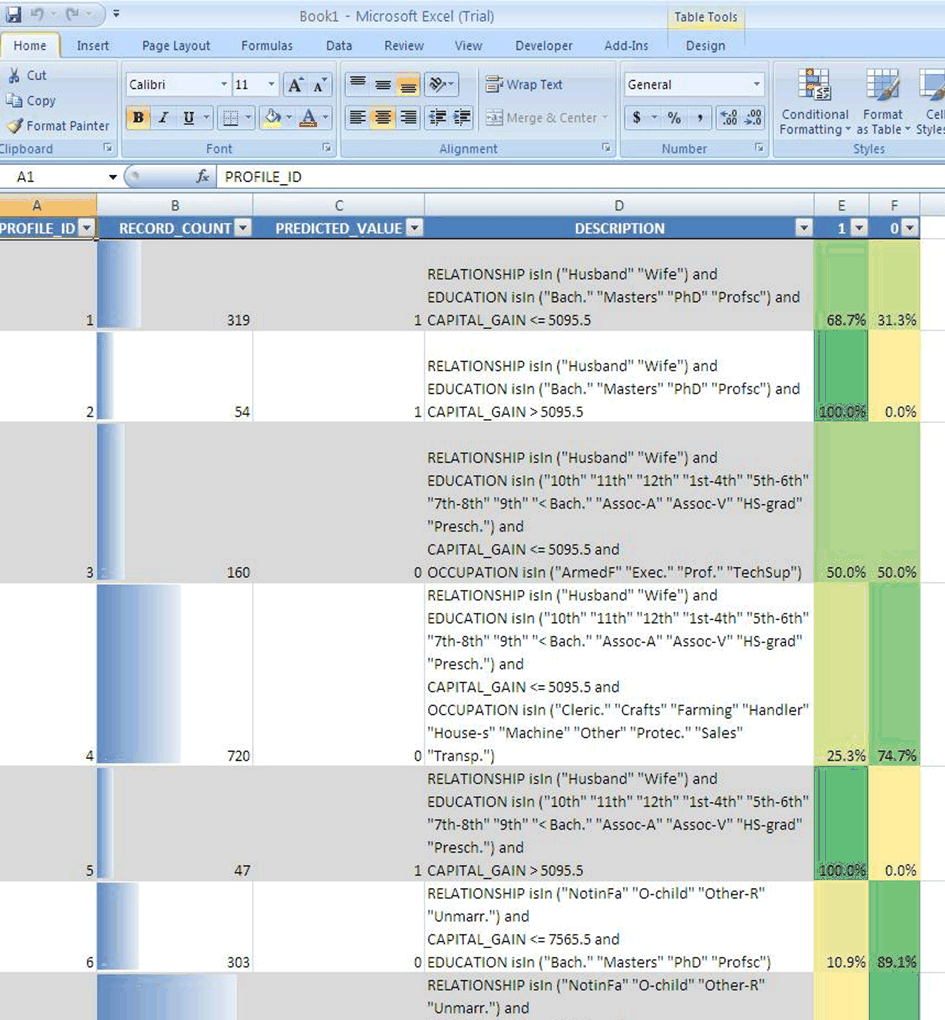# 3Introducing Oracle Predictive Analytics

This chapter presents an overview of Oracle Data Mining predictive analytics, an automated form of predictive data mining.

This chapter includes the following sections:

Predictive Analytics is a technology that captures data mining processes in simple routines. Sometimes called "one-click data mining," predictive analytics simplifies and automates the data mining process.

Predictive analytics develops profiles, discovers the factors that lead to certain outcomes, predicts the most likely outcomes, and identifies a degree of confidence in the predictions.

### Predictive Analytics and Data Mining

Predictive analytics uses data mining technology, but knowledge of data mining is not needed to use predictive analytics.

You can use predictive analytics simply by specifying an operation to perform on your data. You do not need to create or use mining models or understand the mining functions and algorithms summarized in Chapter 2 of this manual.

### How Does it Work?

The predictive analytics routines analyze the input data and create mining models. These models are trained and tested and then used to generate the results returned to the user. The models and supporting objects are not preserved after the operation completes.

When you use data mining technology directly, you create a model or use a model created by someone else. You apply the model to new data (different from the data used to train and test the model). Predictive analytics routines apply the model to the same data used for training and testing.

"Behind the Scenes" to gain insight into the inner workings of Oracle predictive analytic

### Predictive Analytics Operations

Oracle Data Mining predictive analytics operations are described in Table 3-1.

Table 3-1 Oracle Predictive Analytics Operations

Operation Description

`EXPLAIN`

Explains how the individual attributes affect the variation of values in a target column

`PREDICT`

For each case, predicts the values in a target column

`PROFILE`

Creates a set of rules for cases that imply the same target value

The Oracle Spreadsheet Add-In for Predictive Analytics provides predictive analytics operations within a Microsoft Excel spreadsheet. You can analyze Excel data or data that resides in an Oracle database.

Figure 3-1 shows the `EXPLAIN` operation using Microsoft Excel 7.0.Description of "Figure 3-1 EXPLAIN in Oracle Spreadsheet Add-In for Predictive Analytics"

Figure 3-2 shows the `PREDICT` operation.Description of "Figure 3-2 PREDICT in Oracle Spreadsheet Add-In for Predictive Analytics"

Figure 3-3 shows the `PROFILE` operation.Description of "Figure 3-3 PROFILE in Oracle Spreadsheet Add-In for Predictive Analytics"

`http://www.oracle.com/technology/products/bi/odm/`

## APIs for Predictive Analytics

Oracle Data Mining implements predictive analytics in the PL/SQL and Java APIs.

### Predictive Analytics in the PL/SQL API

`DBMS_PREDICTIVE_ANALYTICS` package. The following SQL `DESCRIBE` shows the predictive analytics procedures with their parameters.

```SQL> describe dbms_predictive_analytics

PROCEDURE EXPLAIN
Argument Name                  Type                    In/Out Default?
------------------------------ ----------------------- ------ --------
DATA_TABLE_NAME                VARCHAR2                IN
EXPLAIN_COLUMN_NAME            VARCHAR2                IN
RESULT_TABLE_NAME              VARCHAR2                IN
DATA_SCHEMA_NAME               VARCHAR2                IN     DEFAULT

PROCEDURE PREDICT
Argument Name                  Type                    In/Out Default?
------------------------------ ----------------------- ------ --------
ACCURACY                       NUMBER                  OUT
DATA_TABLE_NAME                VARCHAR2                IN
CASE_ID_COLUMN_NAME            VARCHAR2                IN
TARGET_COLUMN_NAME             VARCHAR2                IN
RESULT_TABLE_NAME              VARCHAR2                IN
DATA_SCHEMA_NAME               VARCHAR2                IN     DEFAULT

PROCEDURE PROFILE
Argument Name                  Type                    In/Out Default?
------------------------------ ----------------------- ------ --------
DATA_TABLE_NAME                VARCHAR2                IN
TARGET_COLUMN_NAME             VARCHAR2                IN
RESULT_TABLE_NAME              VARCHAR2                IN
DATA_SCHEMA_NAME               VARCHAR2                IN     DEFAULT
```

### Predictive Analytics in the Java API

The Oracle Data Mining Java API defines predictive analytics tasks that use the `DBMS_PREDICTIVE_ANALYTICS` package.

The create methods that are defined under `oracle.dmt.jdm.task.OraPredictiveAnalyticsTaskFactory` class are shown as follows:

```public OraPredictTask createPredictTask(String inputDataURI, String caseID,
String targetColumn,
String predictionResultTableName)
throws JDMException;
String explainColumn,
String explainResultTableName)
throws JDMException;
String targetAttributeName,
String profileResultTableName)
throws JDMException;
```

#### Example: Use OraProfileTask to Create Profile Results

```//Step-1: Create OraPredictiveAnalyticsTaskFactory

//Step-2: Create, save and execute OraProfileTask. After
"PROFILE_OUTPUT_JDM");
```

## Example: PREDICT

Example 3-1 shows how a simple `PREDICT` operation can be used to find the customers most likely to increase spending if given an affinity card.

The customer data, including current affinity card usage and other information such as gender, education, age, and household size, is stored in a view called `MINING_DATA_APPLY_V`. The results of the `PREDICT` operation are written to a table named `p_result_tbl`.

The `PREDICT` operation calculates both the prediction and the accuracy of the prediction. Accuracy, also known as predictive confidence, is a measure of the improvement over predictions that would be generated by a naive model. In the case of classification, a naive model would always guess the most common class. In Example 3-1, the improvement is almost 50%.

Example 3-1 Predict Customers Most Likely to Increase Spending with an Affinity Card

```DECLARE
p_accuracy NUMBER(10,9);
BEGIN
DBMS_PREDICTIVE_ANALYTICS.PREDICT(
accuracy                => p_accuracy,
data_table_name         =>'mining_data_apply_v',
case_id_column_name     =>'cust_id',
target_column_name      =>'affinity_card',
result_table_name       =>'p_result_tbl');
DBMS_OUTPUT.PUT_LINE('Accuracy: ' || p_accuracy);
END;
/

Accuracy: .492433267
```

The following query returns the gender and average age of customers most likely to respond favorably to an affinity card.

```SELECT cust_gender, COUNT(*) as cnt, ROUND(AVG(age)) as avg_age
FROM mining_data_apply_v a, p_result_tbl b
WHERE a.cust_id = b.cust_id
AND b.prediction = 1
GROUP BY a.cust_gender
ORDER BY a.cust_gender;

C        CNT    AVG_AGE
- ---------- ----------
F         90         45
M        443         45
```

## Behind the Scenes

This section provides some high-level information about the inner workings of Oracle predictive analytics. If you know something about data mining, you will find this information to be straight-forward and easy to understand. If you are unfamiliar with data mining, you can skip this section. You do not need to know this information to use predictive analytics.

Chapter 2 for an overview of model functions and algorithms

### EXPLAIN

`EXPLAIN` creates an attribute importance model. Attribute importance uses the Minimum Description Length algorithm to determine the relative importance of attributes in predicting a target value. `EXPLAIN` returns a list of attributes ranked in relative order of their impact on the prediction. This information is derived from the model details for the attribute importance model.

Attribute importance models are not scored against new data. They simply return information (model details) about the data you provide.

Attribute importance is described in "Attribute Importance".

### PREDICT

`PREDICT` creates a Support Vector Machine (SVM) model for classification or regression.

`PREDICT` creates a Receiver Operating Characteristics (ROC) curve to analyze the per-case accuracy of the predictions. `PREDICT` optimizes the probability threshold for binary classification models. The probability threshold is the probability that the model uses to make a positive prediction. The default is 50%.

#### Accuracy

`PREDICT` returns a value indicating the accuracy, or predictive confidence, of the prediction. The accuracy is the improvement gained over a naive prediction. For a categorical target, a naive prediction would be the most common class, for a numerical target it would be the mean. For example, if a categorical target can have values `small`, `medium`, or `large`, and `small` is predicted more often than `medium` or `large`, a naive model would return `small` for all cases. Predictive analytics uses the accuracy of a naive model as the baseline accuracy.

The accuracy metric returned by `PREDICT` is a measure of improved maximum average accuracy versus a naive model's maximum average accuracy. Maximum average accuracy is the average per-class accuracy achieved at a specific probability threshold that is greater than the accuracy achieved at all other possible thresholds.

SVM is described in Chapter 18.

### PROFILE

`PROFILE` creates a Decision Tree model to identify the characteristics of the attributes that predict a common target. For example, if the data has a categorical target with values `small`, `medium`, or `large`, `PROFILE` would describe how certain attributes typically predict each size.

The Decision Tree algorithm creates rules that describe the decisions that affect the prediction. The rules, expressed in XML as if-then-else statements, are returned in the model details. `PROFILE` returns XML that is derived from the model details generated by the algorithm.

Decision Tree is described in Chapter 11.